• +91 9971497814
• info@interviewmaterial.com

# RD Chapter 3- Functions Ex-3.3 Interview Questions Answers

### Related Subjects

Question 1 :
Find the domain of each of the following real valued functions of real variable:
(i) f (x) = 1/x
(ii) f (x) = 1/(x-7)
(iii) f (x) = (3x-2)/(x+1)
(iv) f (x) = (2x+1)/(x2-9)
(v) f (x) = (x2+2x+1)/(x2-8x+12)

(i) f(x) = 1/x

We know, f (x) is defined for all real values of x, except for the casewhen x = 0.

Domain of f = R – {0}

(ii) f(x) = 1/(x-7)

We know, f (x) is defined for all real values of x, except for the casewhen x – 7 = 0 or x = 7.

Domain of f = R – {7}

(iii) f(x) = (3x-2)/(x+1)

We know, f(x) is defined for all real values of x, except for the casewhen x + 1 = 0 or x = –1.

Domain of f = R – {–1}

(iv) f(x) = (2x+1)/(x2-9)

We know, f (x) is defined for all real values of x, except for the casewhen x2 – 9 = 0.

x2 – 9 = 0

x2 – 32 = 0

(x + 3)(x – 3) = 0

x + 3 = 0 or x – 3 = 0

x = ± 3

Domain of f = R – {–3, 3}

(v) f(x) = (x2+2x+1)/(x2-8x+12)

We know, f(x) is defined for all real values of x, except for the casewhen x2 – 8x + 12 = 0.

x2 – 8x + 12 = 0

x2 – 2x – 6x + 12 =0

x(x – 2) – 6(x – 2) = 0

(x – 2)(x – 6) = 0

x – 2 = 0 or x – 6 = 0

x = 2 or 6

Domain of f = R – {2, 6}

Question 2 :
Find the domain of each of the following real valued functions of real variable:
(i) f (x) = √(x-2)
(ii) f (x) = 1/(√(x2-1))
(iii) f (x) = √(9-x2)
(iv) f (x) = √(x-2)/(3-x)

(i) f(x) = √(x-2)

We know the square of a real number is never negative.

f (x) takes real values only when x – 2 ≥ 0

x ≥ 2

x  [2,∞)

Domain (f) = [2, ∞)

(ii) f(x) = 1/(√(x2-1))

We know the square of a real number is never negative.

f (x) takes real values only when x2 –1 ≥ 0

x2 – 12 ≥ 0

(x + 1) (x – 1) ≥ 0

x ≤ –1 or x ≥ 1

x  (–∞,–1]  [1, ∞)

In addition, f (x) is also undefined when x2 – 1 = 0 because denominator will be zero and theresult will be indeterminate.

x2 – 1 = 0  x= ± 1

So, x  (–∞, –1]  [1, ∞) – {–1, 1}

(–∞, –1)  (1, ∞)

Domain (f) = (–∞, –1)  (1, ∞)

(iii) f(x) = √(9-x2)

We know the square of a real number is never negative.

f (x) takes real values only when 9 – x2 ≥0

9 ≥ x2

x2 ≤ 9

x2 – 9 ≤ 0

x2 – 32 ≤ 0

(x + 3)(x – 3) ≤ 0

x ≥ –3 and x ≤ 3

[–3, 3]

Domain (f) = [–3, 3]

(iv) f(x) = √(x-2)/(3-x)

We know the square root of a real number is never negative.

f (x) takes real values only when x – 2 and 3 – x are both positive andnegative.

(a) Bothx – 2 and 3 – x are positive

x – 2 ≥ 0

x ≥ 2

3 – x ≥ 0

x ≤ 3

Hence, x ≥ 2 and x ≤ 3

x  [2,3]

(b) Bothx – 2 and 3 – x are negative

x – 2 ≤ 0

x ≤ 2

3 – x ≤ 0

x ≥ 3

Hence, x ≤ 2 and x ≥ 3

However, the intersection of these sets is null set. Thus, this case isnot possible.

Hence, x  [2, 3] – {3}

[2, 3]

Domain (f) = [2, 3]

Question 3 :
Find the domain and range of each of the following real valued functions:
(i) f (x) = (ax+b)/(bx-a)
(ii) f (x) = (ax-b)/(cx-d)
(iii) f (x) = √(x-1)
(iv) f (x) = √(x-3)
(v) f (x) = (x-2)/(2-x)
(vi) f (x) = |x-1|
(vii) f (x) = -|x|
(viii) f (x) = √(9-x2)

(i) f(x) = (ax+b)/(bx-a)

f(x) is defined for all real values of x, except for the case when bx – a= 0 or x = a/b.

Domain (f) = R – (a/b)

Let f (x) = y

(ax+b)/(bx-a) = y

ax + b = y(bx – a)

ax + b = bxy – ay

ax – bxy = –ay – b

x(a – by) = –(ay + b)

x = – (ay+b)/(a-by)

When a – by = 0 or y = a/b

Hence, f(x) cannot take the value a/b.

Range (f) = R – (a/b)

(ii) f(x) = (ax-b)/(cx-d)

f(x) is defined for all real values of x, except for the case when cx – d= 0 or x = d/c. Domain (f) = R – (d/c)

Let f (x) = y

(ax-b)/(cx-d) = y

ax – b = y(cx – d)

ax – b = cxy – dy

ax – cxy = b – dy

x(a – cy) = b – dy

x = (b-dy)/(a-cy)

When a – cy = 0 or y = a/c,

Hence, f(x) cannot take the value a/c.

Range (f) = R – (a/c)

(iii) f(x) = √(x-1)

We know the square of a real number is never negative.

f(x) takes real values only when x – 1 ≥ 0

x ≥ 1

x  [1,∞)

Thus, domain (f) = [1, ∞)

When x ≥ 1, we have x – 1 ≥ 0

Hence, √(x-1) ≥ 0 f (x) ≥ 0

f(x)  [0, ∞)

Range (f) = [0, ∞)

(iv) f(x) = √(x-3)

We know the square of a real number is never negative.

f (x) takes real values only when x – 3 ≥ 0

x ≥ 3

x  [3,∞)

Domain (f) = [3, ∞)

When x ≥ 3, we have x – 3 ≥ 0

Hence, √(x-3) ≥ 0 f (x) ≥ 0

f(x)  [0, ∞)

Range (f) = [0, ∞)

(v) f(x) = (x-2)/(2-x)

f(x) is defined for all real values of x, except for the case when 2 – x =0 or x = 2.

Domain (f) = R – {2}

We have, f (x) = (x-2)/(2-x)

f (x) = -(2-x)/(2-x)

= –1

When x ≠ 2, f(x) = –1

Range (f) = {–1}

(vi) f(x) = |x-1|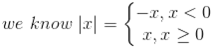Now we have,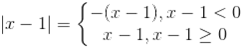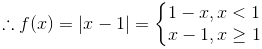Hence, f(x) is defined for all real numbers x.

Domain (f) = R

When, x < 1, we have x – 1 < 0 or 1 – x > 0.

|x – 1| > 0  f(x) > 0

When, x ≥ 1, we have x – 1 ≥ 0.

|x – 1| ≥ 0  f(x) ≥ 0

f(x)≥ 0 or f(x)  [0, ∞)

Range (f) = [0, ∞)

(vii) f(x) = -|x|Now we have,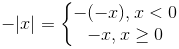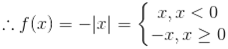Hence, f(x) is defined for all real numbers x.

Domain (f) = R

When, x < 0, we have –|x| < 0

f (x) < 0

When, x ≥ 0, we have –x ≤ 0.

–|x| ≤ 0  f (x) ≤ 0

f(x) ≤ 0 or f (x)  (–∞, 0]

Range (f) = (–∞, 0]

(viii) f(x) = √(9-x2)

We know the square of a real number is never negative.

f(x) takes real values only when 9 – x2 ≥0

9 ≥ x2

x2 ≤ 9

x2 – 9 ≤ 0

x2 – 32 ≤ 0

(x + 3)(x – 3) ≤ 0

x ≥ –3 and x ≤ 3

x  [–3,3]

Domain (f) = [–3, 3]

When, x  [–3, 3], we have 0 ≤ 9 – x2 ≤ 9

0 ≤ √(9-x2) ≤ 3 0 ≤ f (x) ≤ 3

f(x)  [0,3]

Range (f) = [0, 3]

Todays Deals### RD Chapter 3- Functions Ex-3.3 Contributorskrishan

Name:
Email:

# Latest News# 9000 interview questions in different categories